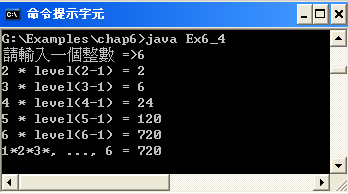Java 程式設計(一)  ：第 六章 方法與套件引用6-4 遞迴函數

• 6-4-1 遞迴函數的流程

• 6-4-2 範例研討：累乘遞迴函數

• 6-4-3 自我挑戰：曼波舞表演系統6-10 遞迴函數的運作程序

 範例：level(n) = 1 * 2 *3 * 4 *, …, *n 說明：total = level(5) 的運作程序 static int level(int k) { if (k <=1) return 1; else return (k* level(k-1)); }6-1 遞迴函數 level(5) 執行步驟

 次數 level(k) K K <=1 執行動作 返回 1 level(5) 5 no return (5 * level(4)) 5 * 24 = 120 2 level(4) 4 no return (4 * level(3)) 4 * 6 = 24 3 level(3) 3 no return ( 3 * level(2))) 3 * 2 = 6 4 level(2) 2 no return (2 * level(1)) 2*1 = 2 5 level(1) 1 yes return 1 1

A）程式功能：Ex6_4.javaB）製作技巧研討：

C）程式範例：

C）程式範例：

 01 02 03 04 05 06 07 08 09 10 11 12 13 14 15 16 17 18 19 20 21 22 23 24 /Ex6_4.java   import java.util.*; public class Ex6_4 {     public static void main(String args[]) {         Scanner keyin = new Scanner(System.in);         int total, num;         System.out.printf("請輸入一個整數 =>");         num = keyin.nextInt();         total = level(num);         System.out.printf("1*2*3*, ..., %d = %d\n", num, total);     }     /* 遞迴函數開始 */     static int level(int k) {         int sum;         if (k <= 1)             return 1;         else {             sum = k * level(k-1);             System.out.printf("%d * level(%d-1) = %d\n", k, k, sum);             return sum;         }     } /* 遞迴函數結束 */ }

(D) 程式重點說明：

(1) 14~23 static int level(int k) {…}。遞迴函數主體。

(2) 16~17 if( k<=1 ) retrun 1;。表示遞迴函數結束。

(3) 18~22 else { sum = k * level(k-1); …}。函數呼叫與自己名稱相同的函數，但引數減少（k-1）。

(4) 20 System.out.printf(…)。印出每次呼叫函數的執行結果。

A）程式功能：PM6-2.java

 G:\Examples\chap6>java PM6_2 == 曼波舞步表演系統 == 請輸入表演級數 =>4 第 1 階舞步 =>1 1 第 2 階舞步 =>1 2 2 1 第 3 階舞步 =>1 2 3 3 2 1 第 4 階舞步 =>1 2 3 4 4 3 2 1 謝謝參觀

B）製作技巧提示。

 觀眾選擇觀賞級數（n）； for (int k=1; k<=n; k++) { 顯示表演階層（k）； 呼叫前進遞迴函數（front_dance(k)）； 呼叫後退遞迴函數（back_dance(k)）； } static void fornt_dance(int k) {     // 前進遞迴函數宣告   if (k <= 1)        System.out.printf("1 ");   else {        front_dance(k-1);        System.out.printf("%d ", k);    } } static void back_dance(int k) {      // 後退遞迴函數宣告    if (k <= 1)         System.out.printf("1 ");    else {         System.out.printf("%d ", k);         back_dance(k-1);    } }

Java 程式設計(一) 含程式邏輯